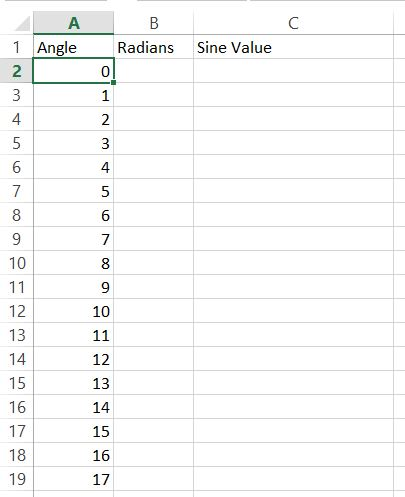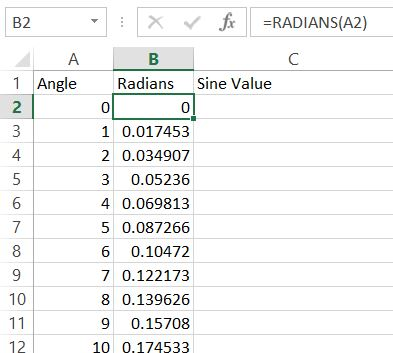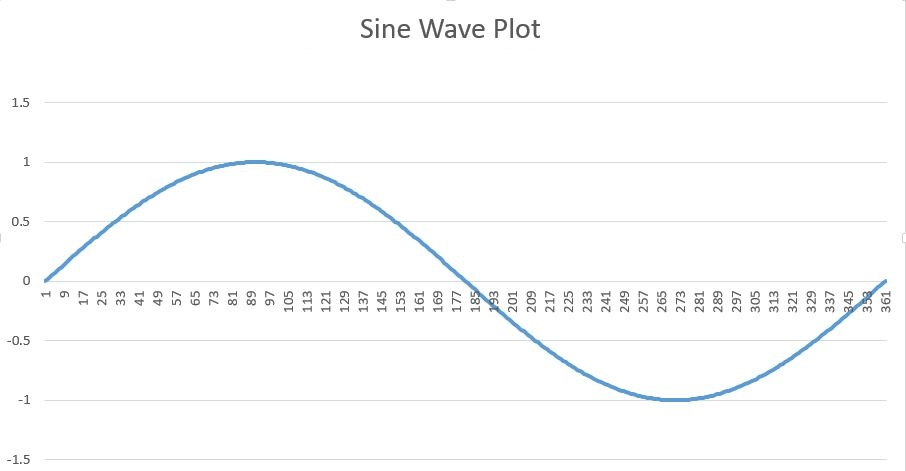### How To Graph A Sine Wave In Excel

In this Excel tutorial you will teach yourself how to graph a sine wave.

To create such sine wave chart first what you need is to prepare data.

Start from writing angles from 0 to 360 degrees.Next you need to convert degrees to radians. To do that just use =RADIANS(A2) and drag down.Next thing you need to do is convert radians to sine value.

Here’s the formula for you. Use Excel SIN function.

Sin formula will be =SIN(B2). Drag it down again. Base on sine values you will be able to insert sine wave plot.Base on sine values create line chart.This is how your sine wave plot looks like. The wave looks nice.

Here’s where you can

## Template

```Further reading: